## ML Aggarwal Class 6 Solutions for ICSE Maths Chapter 15 Data Handling Ex 15.2

Question 1.
Following pictograph shows the number of tractors in five villages.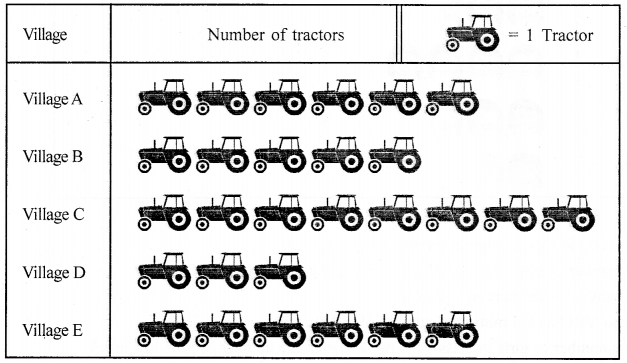Observe the pictograph and answer the following questions.
(i) Which village has the minimum number of tractors?
(ii) Which village has the maximum number of tractors?
(iii) How many more tractors village C has as compared to village B.
(iv) What is the total number of tractor in all the five villages?
Solution:
(i) Village D has the minimum number of tractors.
(ii) Village C has the maximum number of tractors.
(iii) Village C has 8-5 = 3 more tractors as compared to village B.
(iv) Total number of tractors in all the five villages = 6 + 5 + 8 + 3 + 6 = 28.

Question 2.
The number of girl students in each class of a co-educational middle school is depicted by the pictograph.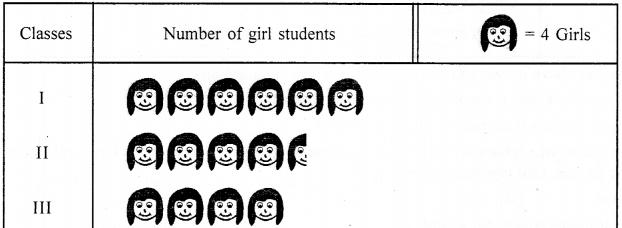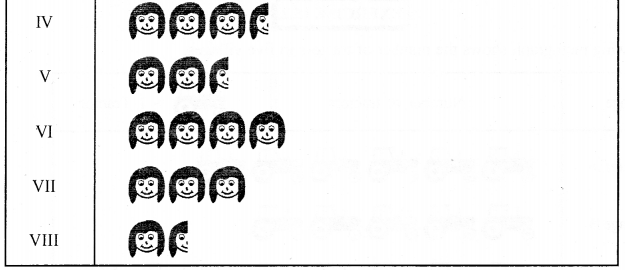Observe this pictograph and answer the following questions :
(a) Which class has the minimum number of girl students?
(b) Is the number of girls in Class VI less than the number of girls in Class V?
(c) How many girls are there in Class VII?
Solution:
(a) Class VIII has a minimum number of girl students.
(b) No! the number of girls in class VI is not less than
the number of girls in Class V.
(c) Number of girls in Class VII = 3 × 4 = 12.

Question 3.
In a village, the following pictograph shows the number of fruit baskets sold by six merchants in a particular season: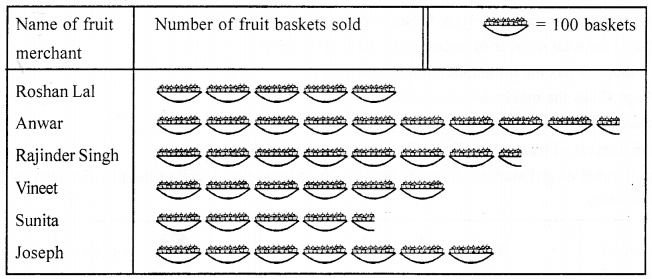Observe the above pictograph and answer the following questions:
(i) Which merchant sold the maximum number of baskets?
(ii) How many baskets were sold by Rajinder Singh?
(iii) The merchants who have sold 700 or more number of baskets are planning to buy a cold store for the next season. Can you name them?
Solution:
(i) Anwar
(ii) 750
(iii) Anwar, Rajinder Singh and Joseph.

Question 4.
In Gurgaon, the number of cars sold during a particular week was as follows:Prepare a pictograph of the cars sold using a symbol of carrepresenting 10 cars and answer the following questions:
(i) On which day the maximum number of cars were sold?
(ii) How many pictures of cars will represent the number of cars sold on Thursday?
Solution: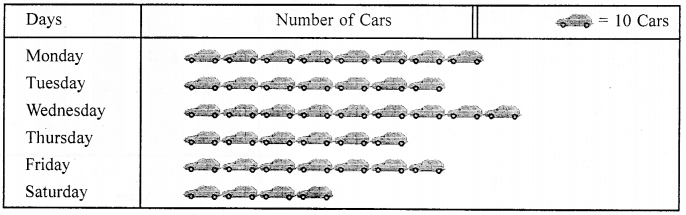(i) Wednesday
(ii) 6

Question 5.
Total number of animals in five villages are as follows: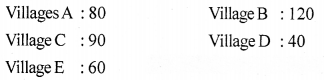Prepare a pictograph of these animals using one symbol ® to represent 10 animals and answer the following questions:
(i) How many symbols represent animals of village E?
(ii) Which village has the maximum number of animals?
(iii) Which village has more animals: village A or village C?
Solution: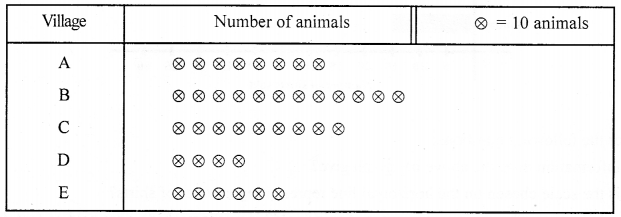(i) 6
(ii) Village B
(iii) Village C